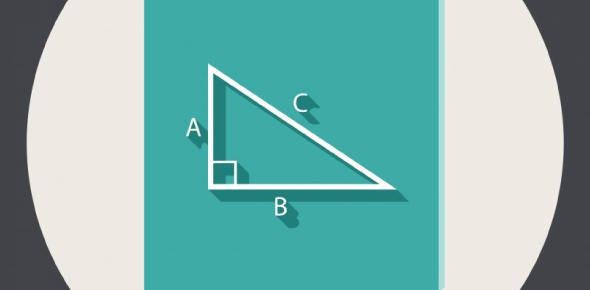Which of the following cannot be X? A triangle has sides of length 7, - ProProfs Discuss

# Which of the following cannot be X? A triangle has sides of length 7, 11, and X.Change Image    Delete

Asked by Harrison, Last updated: Jul 23, 2018

A. 2
B. 4
C. 8
D. 12
E. 18

This question is part of

SAT Math Questions I

### Request AnswerShare... Answer AnonymouslyAnswer Later Copy Link#### AnnJ

AnnJ

18 is also impossible, because the sum of two lenghts cannot be equal with the third, that means that the angle between sides 7 and 11 is 180, and that makes it... a line.Reply#### amsr

Amsr

Replied on Jul 25, 2017

If you look at it that way, neither can be 4, since 7+4=11. Possible answers are 4, 2 and 18ReplyThe answer is clearly 2- if one side is 11 and the other is 7 the 3rd side must be long enough to reach look at the possible length of the 3rd side- if the 7 is on one side of the 11 side and the x is on the other if x were 2 then even with angels of 0 degrees the 7 side would not connect with the x side
​to make a triangle the sum of the 2 smaller sides must equal more than the largest side
7+2 <11ReplyThere are two answers. Not only can the two sides not only be smaller than another than the other they also can not be equal when added together therefore 18 is also correct because 7+11=18ReplyX=4 & x=18 are other values along with x=2 which are not possibleReply#### John Smith

John SmithAnswered on Sep 11, 2016

2
The sum of lengths of two sides of a triangle cannot be less than the length of the third side. So, 2 + 7 = 9, but 9 < 11Reply#### Iskimotu

Iskimotu

18 is impossible too if you define what a triangle must have an acreage.ReplyX=4 & x=18 both are also not possibleReplyA triangle will not be a triangle if the sum of one side is the same or greater than the sum of the other two sides there fore it must be 18.ReplySearch for Google imagesSelect a recommended image
Upload from your computerCancelSearch for Google imagesSelect a recommended image
Upload from your computerCancelSearch for Google imagesSelect a recommended image
Upload from your computerCancel